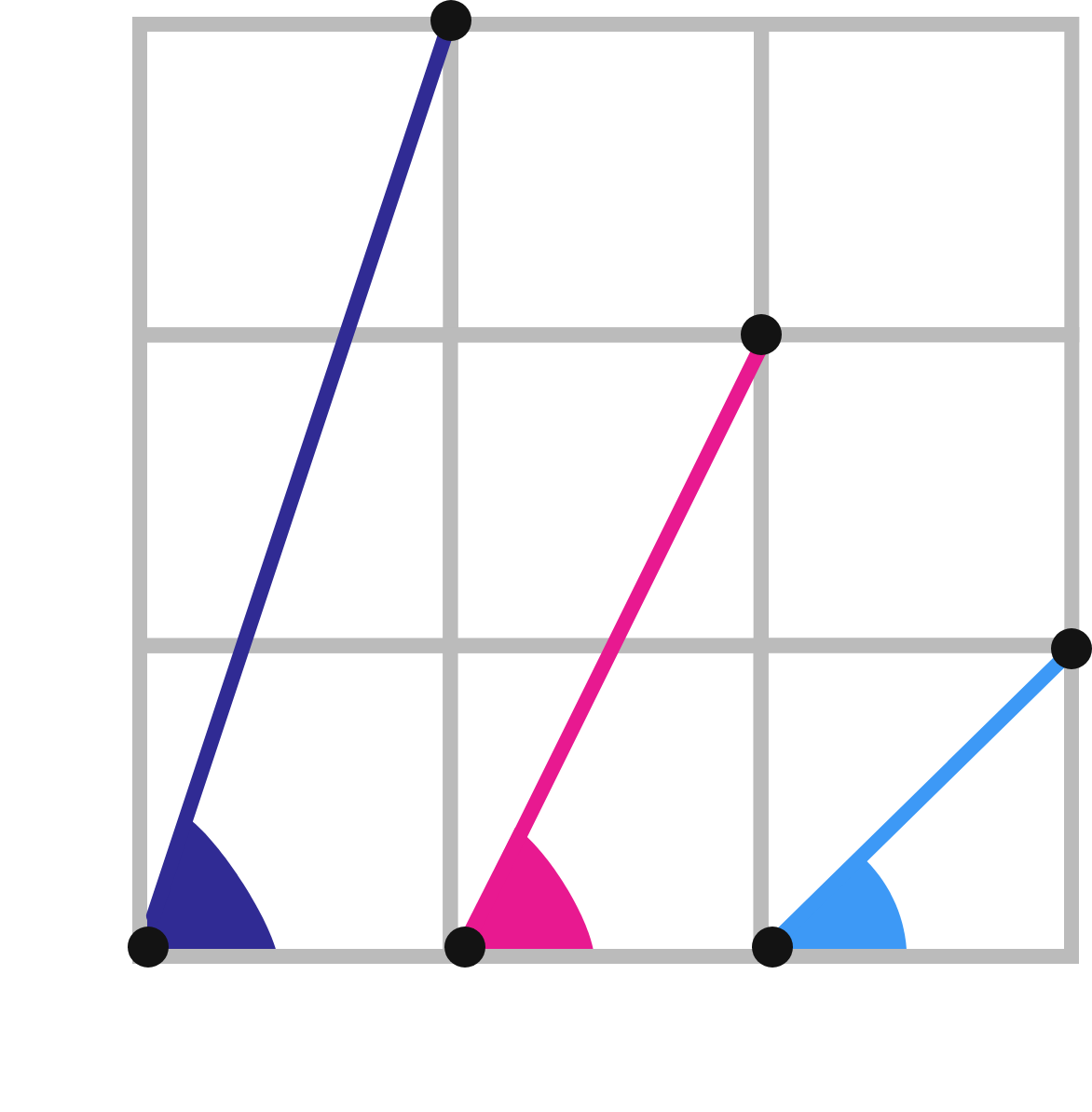# 69 of 100: No Trigonometry Required!

Geometry Level 1The big square above is divided into nine congruent squares. What is the sum of the measures of the three shaded angles?As usual for the geometry problems in this challenge, there are many ways to approach this problem. For example, there's one clever technique that involves shifting the segments to different positions on the grid.

×